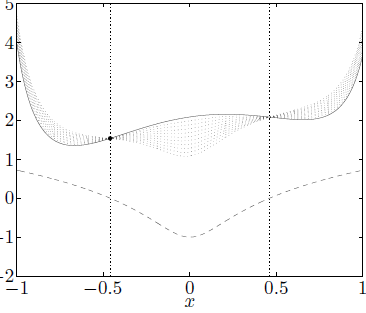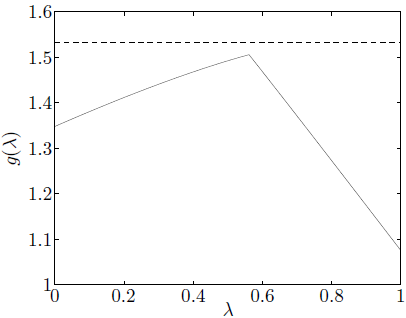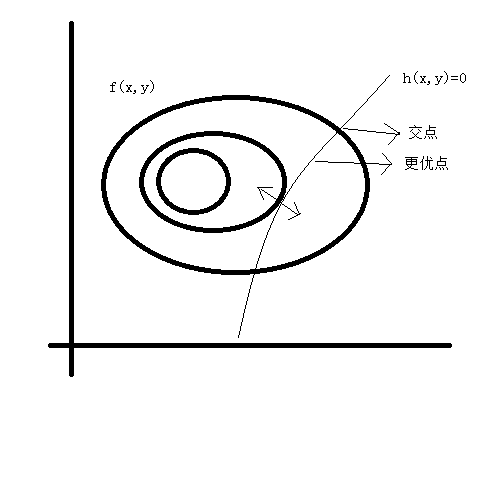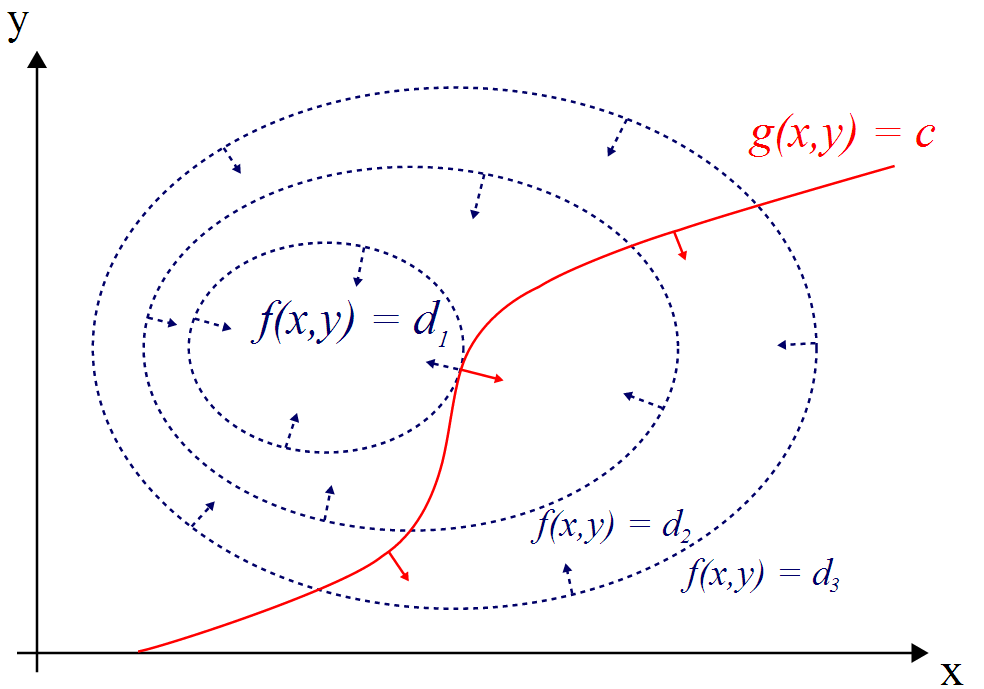kkt 机器学习_机器之心 kkt - CSDN
• 概念：KTT条件是指在满足一些有规则的条件下, 一个非线性规划(Nonlinear Programming)问题能有最优化解法的一个必要和充分条件. 这是一个广义化拉格朗日乘数的成果. 一般地, 一个最优化数学模型的列标准形式参考开头...
概念：

min.:f(x)
s.t.:gi(x)≤0,i=1,2,…,p,
hj(x)=0,k=1,2,…,q,
x∈Ω⊂Rn KTT条件是指在满足一些有规则的条件下, 一个非线性规划(Nonlinear Programming)问题能有最优化解法的一个必要和充分条件. 这是一个广义化拉格朗日乘数的成果. 一般地, 一个最优化数学模型的列标准形式参考开头的式子, 所谓 Karush-Kuhn-Tucker 最优化条件，就是指上式的最优点x∗必须满足下面的条件:
- 1. 约束条件满足gi(x∗)≤0,i=1,2,…,p, 以及,hj(x∗)=0,j=1,2,…,q
- 2. ∇f(x∗)+∑i=1pμi∇gi(x∗)+∑j=1qλj∇hj(x∗)=0, 其中∇为梯度算子;
- 3.  λj≠0且不等式约束条件满足μi≥0,μigi(x∗)=0,i=1,2,…,p

解释：

KKT条件第一项是说最优点x∗必须满足所有等式及不等式限制条件, 也就是说最优点必须是一个可行解, 这一点自然是毋庸置疑的.
第二项表明在最优点x∗, ∇f必须是∇gi和∇hj的线性組合, μi和λj都叫作拉格朗日乘子. 所不同的是不等式限制条件有方向性, 所以每一个μi都必须大于或等于零, 而等式限制条件没有方向性，所以λj没有符号的限制, 其符号要视等式限制条件的写法而定.

展开全文机器学习
• KKT条件(几何的解释)对于凸优化，KKT条件的点就是其极值点(可行下降方向)。 设x∗x^*是非线性规划的局部最小点，目标函数f(x)f(x)在x∗x^*可微，约束方程(g(x))在x∗x^*处可微，连续；则X*点不存在可行下降方向(因为...
KKT条件(几何的解释)

对于凸优化，KKT条件的点就是其极值点(可行下降方向)。

设x∗<!--//--><![CDATA[//><!--
x^*
//--><!]]>是非线性规划的局部最小点，目标函数f(x)<!--//--><![CDATA[//><!--
f(x)
//--><!]]>在x∗<!--//--><![CDATA[//><!--
x^*
//--><!]]>可微，约束方程(g(x))在x∗<!--//--><![CDATA[//><!--
x^*
//--><!]]>处可微，连续；则X*点不存在可行下降方向(因为已经是局部最小点了)
若极小值点在内部，则无约束问题，直接拉格朗日乘子法
若极小值在边界上，(gi(x∗)=0<!--//--><![CDATA[//><!--
g_i(x^*)=0
//--><!]]>)
互补松弛条件 ▽f(x∗)−γ1▽g1(x∗)−γ2▽g2(x∗)=0<!--//--><![CDATA[//><!--
\triangledown f(x^*) -\gamma_1 \triangledown_{g1}(x^*)-\gamma_2 \triangledown_{g2}(x^*)=0
//--><!]]>不起作用的约束包含γi≥0；γigi(x∗)=0<!--//--><![CDATA[//><!--
//--><!]]>
满足其他约束
一阶得到是局部极值点 ，还需要通过二阶判断是否是鞍点
强对偶条件( 鞍点解释)->对偶函数取下确界，则对偶函数一定是凹函数

原函数不好求，转换为求解对偶函数，则对偶函数下确界求得，则比为凹函数（负号为凸函数)
上确界求得，比为凸函数
满足KKT条件后，对偶函数和原函数最优值相等
求解对偶函数，及求解凸函数
对偶和原函数相等（对偶间隔=0）需要满足的式子也是KKT，同时最优点是鞍点，也就是KKT方程的解对偶问题：若要对偶函数的最大值即为原问题的最小值问题(对偶间隙是0)，解凸优化问题等价于解KKT方程；

f0(x∗)=g(λ∗,ν∗)−>（拉格朗日对偶函数）<!--//--><![CDATA[//><!--
f_0(x^* )= g(\lambda ^ *,\nu^*)->（拉格朗日对偶函数）
//--><!]]>=infx(f0(x)+∑i=1mλ∗ifi(x)+∑i=1pvihi(x)<!--//--><![CDATA[//><!--
=\inf\limits_x(f_0(x)+\sum\limits_{i=1}^m\lambda_i^*f_i(x)+\sum\limits_{i=1}^pv_ih_i(x)
//--><!]]>≤infx(f0(x)+∑i=1mλ∗ifi(x)+∑i=1pvihi(x)<!--//--><![CDATA[//><!--
\leq \inf\limits_x(f_0(x)+\sum\limits_{i=1}^m\lambda_i^*f_i(x)+\sum\limits_{i=1}^pv_ih_i(x)
//--><!]]>≤f0(x∗)<!--//--><![CDATA[//><!--
\leq f_0(x^*)
//--><!]]>
上式等号成立需要：

fi(x∗)≤0,i=1,...,m<!--//--><![CDATA[//><!--
f_i(x^*) \leq 0, i=1,...,m
//--><!]]>hi(x∗)=0,i=1,...,m<!--//--><![CDATA[//><!--
h_i(x^*) = 0, i=1,...,m
//--><!]]>λ∗i≥0,i=1,...,m<!--//--><![CDATA[//><!--
\lambda_i^* \geq 0, i=1,...,m
//--><!]]>λ∗ifi(x∗)=0,i=1,...,m<!--//--><![CDATA[//><!--
\lambda_i^*f_i(x^*)=0, i=1,...,m
//--><!]]>▽f0(x∗)+∑i=1mλ∗i▽fi(x∗)+∑i=1pvi▽hi(x∗)=0<!--//--><![CDATA[//><!--
\triangledown f_0(x^*)+\sum\limits_{i=1}^m\lambda_i^*\triangledown f_i(x^*)+\sum\limits_{i=1}^pv_i\triangledown h_i(x^*)=0
//--><!]]>
展开全文• 要深入理解SVM算法必需深入理解KKT条件，本文尝试使用简单易懂的方法向读者介绍KKT条件的推导和使用方法。博主尽量使用图形来阐述KKT条件的深层内涵，数学功底较弱的读者可直接跳过推导过程看结论和
前言

本文旨在详细介绍KKT条件的推导和计算方法。
拉格朗日算子常用语等式约束最优化的求解中，是KKT条件的特殊形式。KKT条件用于含有不等式约束的条件下的优化问题，例如SVM算法。要深入理解SVM算法必需深入理解KKT条件，本文尝试使用简单易懂的方法向读者介绍KKT条件的推导和使用方法。博主尽量使用图形来阐述KKT条件的深层内涵，数学功底较弱的读者可直接跳过推导过程看结论和例子。

拉格朗日乘子法

考虑如下约束条件下的：
minxf(x)s.t.hi(x)=0i=1,2,⋯k\begin{array}{l}
\end{array}
其中：
x∈Rnx \in {\Re ^n}
本博客以二元函数为例介绍拉格朗日乘子法的推导过程。
考虑如下优化问题：f(x,y)$f({x},{y})$满足约束h(x,y)=0$h({x},{y}) = 0$
如果在(x0,y0)$(x_0,y_0)$处取得极值，那么有:
h(x0,y0)=0h(x_0,y_0) = 0
约束条件确定一个连续且具有连续导数的函数：
y=φ(x){y} = \varphi ({x})
这时，原问题为：
f(x,φ(x))f({x},\varphi ({x}))
上式在(x0,y0)$(x_0,y_0)$处取得极值，由一元可导函数取得极值的必要条件知道：
fx(x0,y0)+fy(x0,y0)dydx∣∣∣x=x0=0{f_x}({x_0},{y_0}) + {f_y}({x_0},{y_0}){\left. {\frac{{{\text{d}}y}}{{{\text{d}}x}}} \right|_{x = {x_0}}} = 0
由隐函数求导公式有：
dydx∣∣∣x=x0=−hx(x0,y0)hy(x0,y0){\left. {\frac{{{\text{d}}y}}{{{\text{d}}x}}} \right|_{x = {x_0}}} =  - \frac{{{h_x}({x_0},{y_0})}}{{{h_y}({x_0},{y_0})}}
所以有：
fx(x0,y0)−fy(x0,y0)hx(x0,y0)hy(x0,y0)=0{f_x}({x_0},{y_0}) - {f_y}({x_0},{y_0})\frac{{{h_x}({x_0},{y_0})}}{{{h_y}({x_0},{y_0})}} = 0
引入λ$\lambda$，有：
fx(x0,y0)hx(x0,y0)=fy(x0,y0)hy(x0,y0)=−λ\frac{{{f_x}({x_0},{y_0})}}{{{h_x}({x_0},{y_0})}} = \frac{{{f_y}({x_0},{y_0})}}{{{h_y}({x_0},{y_0})}} =  - \lambda
这样，上述必要条件就变为：
fx(x0,y0)+λhx(x0,y0)=0fy(x0,y0)+λhy(x0,y0)=0h(x0,y0)=0\begin{array}{l}
{f_x}({x_0},{y_0}) + \lambda {h_x}({x_0},{y_0}) = 0\\
{f_y}({x_0},{y_0}) + \lambda {h_y}({x_0},{y_0}) = 0\\
h({x_0},{y_0}) = 0
\end{array}
若引入辅助函数：
L(x,y)=f(x,y)+λh(x,y)L(x,y) = f(x,y) + \lambda h(x,y)
这不难看出，上述极值条件为：
Lx(x,y)=0Ly(x,y)=0Lλ(x,y)=0\eqalign{
& {L_x}(x,y) = 0  \cr
& {L_y}(x,y) = 0  \cr
& {L_\lambda }(x,y) = 0 \cr}
推广到多元情况为：
L(x1,⋯,xn)=f(x1,⋯,xn)+∑ikαihi(x1,⋯,xn)L({x_1}, \cdots ,{x_n}) = f({x_1}, \cdots ,{x_n}) + \sum\limits_i^k {{\alpha _i}{h_i}({x_1}, \cdots ,{x_n})}
L(x1,⋯,xn)$L({x_1}, \cdots ,{x_n})$的极值点即原问题的极值点。
即满足以下条件的点即原问题的最优解：
∇Lxi(x1,⋯,xn)=0 i=1,⋯,n∇Lαj(x1,⋯,xn)=0j=1,⋯,k\eqalign{
& \nabla {L_{{x_i}}}({x_1}, \cdots ,{x_n}) = 0 \qquad\qquad\ i = 1, \cdots ,n  \cr
& \nabla {L_{{\alpha _j}}}({x_1}, \cdots ,{x_n}) = 0 \qquad\qquad j = 1, \cdots ,k \cr}
以下以几何形式阐述这一原理：图中的用等高线表示f(x,y)$f(x,y)$。由于原优化问题是凸优化问题，当h(x,y)=0$h(x,y) = 0$ 和等高线相交时意味着在两个交点之间存在更优的点。只有当等高线和h(x,y)=0$h(x,y) = 0$ 相切时才是满足约束条件的最优点。即在交点处满足：
∇f(x,y)=−λ∇h(x,y)h(x,y)=0\eqalign{
& \nabla f(x,y) =  - \lambda \nabla h(x,y)  \cr
&\;\;\;h(x,y) = 0 \cr}
以下以一例子说明拉格朗日乘子法的计算步骤。

未完待续。。。
/**********************
* 本文来自博客  “zhb_bupt“
* 转载请标明出处:http://blog.csdn.net/zhb_bupt
* 博客迁往： http://deepminer.ailifenet.com
********************************/
展开全文机器学习 算法 svm 优化
• 1.引言本篇博客主要总结了拉格朗日乘子和KTT条件在机器学习中求解最优值的原理，博主尽量举点小例子帮助大家一起共同学习。2.拉格朗日和KTT作用我们在求解问题时，经常会遇到一些在约束条件下求解函数的。 在有等式...
1.引言

本篇博客主要总结了拉格朗日乘子和KTT条件在机器学习中求解最优值的原理，博主尽量举点小例子帮助大家一起共同学习。

2.拉格朗日和KKT作用

我们在求解问题时，经常会遇到一些在约束条件下求解函数的。
在有等式约束条件下，我们选用拉格朗日乘子；
在有不等式约束条件下，选用KKT方法求解最优解。
因此我们可以将KKT条件看成是拉格朗日乘子的泛化。

3.求解最优问题的集中形式

通常我们需要求解的最优化问题有如下几类：

无约束条件下求最优值 minf(x,y)<!--//--><![CDATA[//><!--
minf(x,y)
//--><!]]>
在等式条件下求解最优值 minf(x,y)<!--//--><![CDATA[//><!--
minf(x,y)
//--><!]]>s.t...g(x,y)=c<!--//--><![CDATA[//><!--
s.t... g(x,y)=c
//--><!]]>
在不等式条件下求解最优值 minf(x,y)<!--//--><![CDATA[//><!--
minf(x,y)
//--><!]]>s.t…g(x)<=0,h(x)<=0<!--//--><![CDATA[//><!--
s.t… g(x)<=0
,h(x)<=0
//--><!]]>
4.求解不同最优问题方法

4.1无约束条件下的最优值求解

无约束条件下的函数f(x,y)<!--//--><![CDATA[//><!--
f(x,y)
//--><!]]>求解最大或者最小值，一般求该函数对各个参数的偏导数，令偏导数为0，求解得到的x0,y0<!--//--><![CDATA[//><!--
x_0,y_0
//--><!]]>即为在该条件下可以使函数f(x,y)<!--//--><![CDATA[//><!--
f(x,y)
//--><!]]>最优。 αf(x,y)/αx=0<!--//--><![CDATA[//><!--
\alpha f(x,y)/\alpha x = 0
//--><!]]>αf(x,y)/αy=0<!--//--><![CDATA[//><!--
\alpha f(x,y)/\alpha y = 0
//--><!]]>
这样求解的首先需要保证函数f(x,y)<!--//--><![CDATA[//><!--
f(x,y)
//--><!]]>为凸函数。

4.2 等式条件下的最优值求解（拉格朗日乘子）

假设我们需要求解的函数为f(x,y)<!--//--><![CDATA[//><!--
f(x,y)
//--><!]]>的最优值，同时需要满足条件 g(x,y)=c<!--//--><![CDATA[//><!--
g(x,y)=c
//--><!]]>，因此问题就转化为，在 g(x,y)=c<!--//--><![CDATA[//><!--
g(x,y)=c
//--><!]]>条件下，求解函数f(x,y)<!--//--><![CDATA[//><!--
f(x,y)
//--><!]]>的最优值。函数f(x,y)=d<!--//--><![CDATA[//><!--
f(x,y)=d
//--><!]]>在x,y平面上面具有一些等高线，如下图所示g(x,y)=c<!--//--><![CDATA[//><!--
g(x,y)=c
//--><!]]>是一条红色的曲线，那么我们在什么样的情况下可以求出他的最优值呢，我们发现函数g(x,y)=c<!--//--><![CDATA[//><!--
g(x,y)=c
//--><!]]>会与f(x,y)=d<!--//--><![CDATA[//><!--
f(x,y)=d
//--><!]]>相交，那么交点是不是最优值呢，我们以前知识学过，两个函数的交点说明，是两个函数共同的可行解。那最优点怎样求取呢，我们可以更改d的大小，我们得到一系列的等高线。红色曲线与函数f(x,y)=d<!--//--><![CDATA[//><!--
f(x,y)=d
//--><!]]>的交点就是最优解。
而拉格朗日乘子就是解决这样问题的，通过引入一个λ<!--//--><![CDATA[//><!--
\lambda
//--><!]]>约束条件g(x,y)=c<!--//--><![CDATA[//><!--
g(x,y)=c
//--><!]]>组合得到一个新的函数，这个新函数与目标函数f(x,y)<!--//--><![CDATA[//><!--
f(x,y)
//--><!]]>，得到最终需要优化函数。 L(x,y,λ)=f(x,y)+λ[g(x,y)−c]<!--//--><![CDATA[//><!--
L(x,y,\lambda)=f(x,y)+\lambda[g(x,y)-c]
//--><!]]>
接下来就是求解函数L(x,y,λ)<!--//--><![CDATA[//><!--
L(x,y,\lambda)
//--><!]]>对各个参数的偏导数，并令各个偏导数为0，联立可以求出三个参数x,y,λ<!--//--><![CDATA[//><!--
x,y,\lambda
//--><!]]>,代入原式，可以求解最优解。 αL(x,y,λ)/αx=0<!--//--><![CDATA[//><!--
\alpha L(x,y,\lambda)/\alpha x = 0
//--><!]]>αL(x,y,λ)/αy=0<!--//--><![CDATA[//><!--
\alpha L(x,y,\lambda)/\alpha y = 0
//--><!]]>αL(x,y,λ)/αλ=0<!--//--><![CDATA[//><!--
\alpha L(x,y,\lambda)/\alpha \lambda = 0
//--><!]]>接下来举一个小例子 f(x,y)=x2y<!--//--><![CDATA[//><!--
f(x,y)=x^2y
//--><!]]>,g(x,y)=x2+y2−1<!--//--><![CDATA[//><!--
g(x,y)=x^2+y^2-1
//--><!]]>
则可以得到 L(x,y,λ)=f(x,y)+λg(x,y)=x2y+λ(x2+y2−1)<!--//--><![CDATA[//><!--
L(x,y,\lambda)=f(x,y)+\lambda g(x,y)=x^2y+\lambda (x^2+y^2-1)
//--><!]]>
对各个参数求解偏导数得到 2xy+2λx=0<!--//--><![CDATA[//><!--
2xy+2\lambda x=0
//--><!]]>x2+2λy=0<!--//--><![CDATA[//><!--
x^2+2\lambda y=0
//--><!]]>x2+y2−1=0<!--//--><![CDATA[//><!--
x^2+y^2-1=0
//--><!]]>4.3不等式条件下求解最优解（KKT条件）

对KTT条件的讲解主要采用作者（xianlingmao），对作者表示感谢
对于含有不等式约束的优化问题，如何求取最优值呢？常用的方法是KKT条件，同样地，把所有的不等式约束、等式约束和目标函数全部写为一个式子L(a,b,x)=f(x)+a∗g(x)+b∗h(x)<!--//--><![CDATA[//><!--
L(a, b, x)= f(x) + a*g(x)+b*h(x)
//--><!]]>，KKT条件是说最优值必须满足以下条件：

L(a, b, x)对x求导为零；
h(x) =0;
a*g(x) = 0;
求取这三个等式之后就能得到候选最优值。其中第三个式子非常有趣，因为g(x)<=0<!--//--><![CDATA[//><!--
g(x)<=0
//--><!]]>，如果要满足这个等式，必须a=0<!--//--><![CDATA[//><!--
a=0
//--><!]]>或者g(x)=0<!--//--><![CDATA[//><!--
g(x)=0
//--><!]]>.
KTT满足的强对偶条件可以这样理解，我们要求minf(x)<!--//--><![CDATA[//><!--
min f(x)
//--><!]]>, L(a,b,x)=f(x)+a∗g(x)+b∗h(x)<!--//--><![CDATA[//><!--
L(a, b, x) = f(x) + a*g(x) + b*h(x)
//--><!]]>，a>=0<!--//--><![CDATA[//><!--
a>=0
//--><!]]>，我们可以把f(x)写为：maxa,bL(a,b,x)<!--//--><![CDATA[//><!--
max_{a,b} L(a,b,x)
//--><!]]>，为什么呢？因为h(x)=0,g(x)<=0<!--//--><![CDATA[//><!--
h(x)=0, g(x)<=0
//--><!]]>，现在是取L(a,b,x)<!--//--><![CDATA[//><!--
L(a,b,x)
//--><!]]>的最大值，a*g(x)是<=0，所以L(a,b,x)只有在a*g(x) = 0的情况下才能取得最大值，否则，就不满足约束条件，因此max_{a,b} L(a,b,x)在满足约束条件的情况下就是f(x)，因此我们的目标函数可以写为 min_x max_{a,b} L(a,b,x)。如果用对偶表达式： max_{a,b} min_x  L(a,b,x)，由于我们的优化是满足强对偶的（强对偶就是说对偶式子的最优值是等于原问题的最优值的），所以在取得最优值x0的条件下，它满足 f(x0) = max_{a,b} min_x  L(a,b,x) = min_x max_{a,b} L(a,b,x) =f(x0)，我们来看看中间两个式子发生了什么事情：
f(x0) = max_{a,b} min_x  L(a,b,x) =  max_{a,b} min_x f(x) + a*g(x) + b*h(x) =  max_{a,b} f(x0)+a*g(x0)+b*h(x0) = f(x0)

可以看到上述加黑的地方本质上是说 min_x f(x) + a*g(x) + b*h(x) 在x0取得了最小值，用fermat定理，即是说对于函数 f(x) + a*g(x) + b*h(x)，求取导数要等于零，即

f(x)的梯度+a*g(x)的梯度+ b*h(x)的梯度 = 0

这就是kkt条件中第一个条件：L(a, b, x)对x求导为零。

而之前说明过，a*g(x) = 0，这时kkt条件的第3个条件，当然已知的条件h(x)=0必须被满足，所有上述说明，满足强对偶条件的优化问题的最优值都必须满足KKT条件，即上述说明的三个条件。可以把KKT条件视为是拉格朗日乘子法的泛化。
展开全文拉格朗日乘子 机器学习
• 在求解最优化问题中，拉格朗日乘子法（Lagrange Multiplier）和KKT（Karush Kuhn Tucker）条件是两种最常用的方法。在有等式约束时使用拉格朗日乘子法，在有不等约束时使用KKT条件。　我们这里提到的最优化问题通常...
• 昨天我们介绍了KKT 的大概今天稍微详细的看一下。 KKT理论所标明的是在拉格朗日乘数法中引入的系数与上面的不等式约束条件的乘积等于0始终成立，这个条件所保证的是优化问题的解存在。 下面我们来逐个分析这几个KKT...机器学习
• 学习了一个学期机器学习算法，从什么都不懂到对十个机器学习算法有一定的了解，下面总结一下十大机器学习算法，从算法的概念、原理、优点、缺点、应用等方面来总结，如果有错误的地方，欢迎指出。 目录 1.决策树...
• ## 学好机器学习需要哪些数学知识？

万次阅读 多人点赞 2019-10-21 15:07:14
其它机器学习、深度学习算法的全面系统讲解可以阅读《机器学习-原理、算法与应用》，清华大学出版社，雷明著，由SIGAI公众号作者倾力打造。 书的购买链接 书的勘误，优化，源代码资源 很多同学谈数学色变，但数学...机器学习 数据挖掘
• 机器学习实战》简化版SMO代码中2个判断条件的思考准备工作公式推导代码判断条件欢迎使用Markdown编辑器新的改变功能快捷键合理的创建标题，有助于目录的生成如何改变文本的样式插入链接与图片如何插入一段漂亮的...机器学习 支持向量机 机器学习实战 python
• 上一节讲了带等式约束条件的函数凸优化方法拉格朗日乘子法，本节讲讲带不等式约束条件的函数凸优化方法——KKT条件，为之后深入讲解SVM做准备。机器学习 KKT条件 SVM 支持向量机
• 机器学习无疑是当前数据分析领域的一个热点内容。很多人在平时的工作中都或多或少会用到机器学习的算法。本文为您总结一下常见的机器学习算法，以供您在工作和学习中参考。  机器学习的算法很多。很多时候...机器学习算法汇总 机器学习算法大全 机器学习算法总结
• 有约束的最优化问题 最优化问题一般是指对于某一个函数而言，求解在其指定作用域上的全局最小值问题，一般分为以下三种情况(备注：以下几种方式求出来的解都有可能是局部极小值，只有当函数是凸函数的时候，才可以...
• 机器学习实战》6.2小节 1 #这句是检测 当前样本点i 是否满足KKT条件的 2 if (alphas[i, :] < C and E_i * labelMat[i, :] < -tolerence) or \ 3 (alphas[i, :] > 0 and E_i * labelMat[i,:] > ...
• 机器学习面试问题汇总—史上最详细 BAT机器学习面试1000题系列 特征工程问题汇总 机器学习之特征工程相关问题 模型优化问题 常见的损失函数有哪些？ 机器学习中常见的损失函数 机器学习中防止过...优化算法 深度学习优化算法 机器学习优化算法 Adam SGD
• 对于含有不等式约束的优化问题，可以转化为在满足 KKT 约束条件下应用拉格朗日乘子法求解。拉格朗日求得的并不一定是最优解，只有在凸优化的情况下，才能保证得到的是最优解，所以本文称拉格朗日乘子法得到的为可行...机器学习 计算方法
• 其它机器学习、深度学习算法的全面系统讲解可以阅读《机器学习-原理、算法与应用》，清华大学出版社，雷明著，由SIGAI公众号作者倾力打造。 书的购买链接 书的勘误，优化，源代码资源 《机器学习中的最优化算法...机器学习 人工智能
• 机器学习 凸优化、拉格朗日对偶性和 KKT 条件 凸集 凸函数 凸优化 水平子集 仿射函数 优化 约束优化 拉格朗日对偶性 原始问题 原始问题的拉格朗日函数 原始问题的对偶问题 原始问题与对偶问题的关系 KKT 条件机器学习 凸优化 拉格朗日对偶性 拉格朗日乘子法 KKT 条件
• 机器学习 ...机器学习之 拉格朗日乘子法 和 KKT 机器学习之梯度下降法 GD 机器学习之 MLE 和 MAP 机器学习算法之线性回归 机器学习之 线性回归 接口和代码 机器学习算法之 logistic、Softmax 回归 ...
• ## 机器学习（二）--- 分类算法详解

万次阅读 多人点赞 2015-10-07 15:46:53
感觉狼厂有些把机器学习和数据挖掘神话了，机器学习、数据挖掘的能力其实是有边界的。机器学习、数据挖掘永远是给大公司的业务锦上添花的东西，它可以帮助公司赚更多的钱，却不能帮助公司在与其他公司的竞争中取得...
...